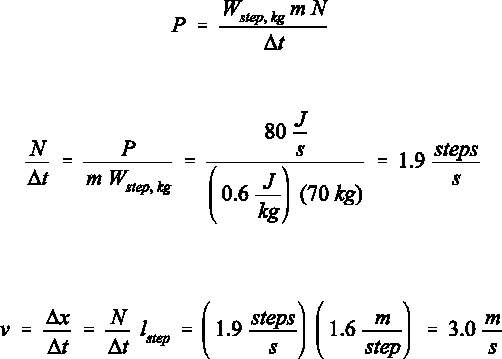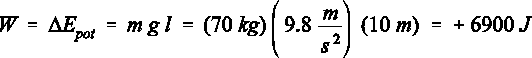# Physics 1028 Lecture 2: Chapter 9

5 views5 pages
School
Western University
Department
Physics
Course
Physics 1028
ProfessorSelection of Even–Numbered Problem Solutions
Chapter 9
P–9.4
The best way to proceed with this problem is to focus on the units of the given parameters. We define as N
the number of steps taken during the time interval t. The work per step and per kilogram of body mass is
Wstep, kg = 0.6 J/kg. Note that "step" is not a standard unit, and is therefore not included in the units. The energy
dissipation P has the unit J/s with P = 80 J/s; this para-meter is a power term.
With the variables defined above we write for the energy dissipation:
(1)
which leads to the number of steps taken per second:
(2)
This number allows us in turn to calculate the speed of the person since we know that each step is 1.6 metres
long:
(3)
Unlock document

This preview shows pages 1-2 of the document.
Unlock all 5 pages and 3 million more documents.P–9.6
When a standard man climbs up a rope, the potential energy of the person changes. To increase the potential
energy of the body, work must be done. The amount of work for a climb of length l is given by:
(4)
The text states that this is only 20% of the total energy required. Thus, the total energy required for the rope
climbing is 34.5 kJ. The heat dissipated in turn is 80% of this value, which is 27.6 kJ.
Where does the energy come from? Remember, the first law of thermodynamics states that energy
cannot be created, it can only be converted from one form into another. The energy in this case is the food
energy stored in the muscles of the person. This also explains why the question is asked the way it is stated.
The term dissipating implies that chemical energy is converted into thermal energy.
It is not unusual, albeit not particularly up to date, to request that the result is stated in a non–standard
unit (here cal). Using the conversion from Joule to calories, we find for the change in the potential energy
Epot = 1.65 kcal, for the total energy required 8.25 kcal and for the dissipated heat 6.6 kcal.
Unlock document

This preview shows pages 1-2 of the document.
Unlock all 5 pages and 3 million more documents.

## Document Summary

The best way to proceed with this problem is to focus on the units of the given parameters. We define as n the number of steps taken during the time interval t. The work per step and per kilogram of body mass is. Note that "step" is not a standard unit, and is therefore not included in the units. The energy dissipation p has the unit j/s with p = 80 j/s; this para-meter is a power term. With the variables defined above we write for the energy dissipation: which leads to the number of steps taken per second: (1) (2) This number allows us in turn to calculate the speed of the person since we know that each step is 1. 6 metres long: (3) When a standard man climbs up a rope, the potential energy of the person changes. To increase the potential energy of the body, work must be done.